Contents Articles Behaviors Books Director News Director Web Sites FAQ Games Mailing Lists News Groups Project Examples Reviews Software Tools Useful Web Sites Utilities Xtras

 Don't miss these#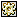Random numbers from file names -w/o BuddyAPI

 Compatibilities: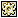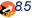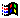This item has not yet been rated

Author: psykopath (website)

Creates random numbers from file names. Does not need the buddy API, but might not work too good on macs, unless their hard drive is named "hard disk". For PC the main drive must be "c:". For script without this limitation see the RNG w/ Buddy API. Format: rand(num, mode) mode: "float" or "integer" Does return 0 so be careful what you use it for.

 on rand lim, mode   --CREATES RANDOM NUMBERS FROM LETTERS IN FILE NAMES ON THE HARD DISK   tString = string(the ticks)   tCount = tString.char.count   tVar = integer( tString.char[tCount] )   tVar2 = integer( tString.char[tCount-1] )   case tVar2 of     1: --set 1 of numbers       case tVar of         0: cFile = 15         1: cFile = 19         2: cFile = 9         3: cFile = 11         4: cFile = 7         5: cFile = 13         6: cFile = 5         7: cFile = 3         8: cFile = 17         9: cFile = 1       end case     2: --set 2 of numbers       case tVar of         0: cFile = 11         1: cFile = 15         2: cFile = 17         3: cFile = 19         4: cFile = 13         5: cFile = 7         6: cFile = 3         7: cFile = 1         8: cFile = 9         9: cFile = 5       end case     3: --set 3 of numbers       case tVar of         0: cFile = 1         1: cFile = 5         2: cFile = 9         3: cFile = 13         4: cFile = 17         5: cFile = 19         6: cFile = 15         7: cFile = 11         8: cFile = 7         9: cFile = 3       end case     4: --set 4 of numbers       case tVar of         0: cFile = 3         1: cFile = 7         2: cFile = 11         3: cFile = 15         4: cFile = 19         5: cFile = 17         6: cFile = 13         7: cFile = 9         8: cFile = 5         9: cFile = 1       end case     5: --set 5 of numbers       case tVar of         0: cFile = 15         1: cFile = 11         2: cFile = 7         3: cFile = 3         4: cFile = 1         5: cFile = 5         6: cFile = 9         7: cFile = 13         8: cFile = 17         9: cFile = 19       end case     6: --set 6 of numbers       case tVar of         0: cFile = 7         1: cFile = 5         2: cFile = 9         3: cFile = 1         4: cFile = 13         5: cFile = 17         6: cFile = 15         7: cFile = 3         8: cFile = 19         9: cFile = 11       end case     7: --set 7 of numbers       case tVar of         0: cFile = 2         1: cFile = 4         2: cFile = 6         3: cFile = 8         4: cFile = 10         5: cFile = 12         6: cFile = 14         7: cFile = 16         8: cFile = 18         9: cFile = 20       end case     8: --set 8 of numbers       case tVar of         0: cFile = 20         1: cFile = 18         2: cFile = 16         3: cFile = 14         4: cFile = 12         5: cFile = 10         6: cFile = 8         7: cFile = 6         8: cFile = 4         9: cFile = 2       end case     9: --set 9 of numbers       case tVar of         0: cFile = 4         1: cFile = 14         2: cFile = 8         3: cFile = 18         4: cFile = 12         5: cFile = 10         6: cFile = 20         7: cFile = 6         8: cFile = 16         9: cFile = 2       end case     0: --set 10 of numbers       case tVar of         0: cFile = 1         1: cFile = 10         2: cFile = 2         3: cFile = 9         4: cFile = 3         5: cFile = 8         6: cFile = 4         7: cFile = 7         8: cFile = 5         9: cFile = 6       end case   end case   if the platform contains "Windows" then myPath = "C:"   if the platform contains "Macintosh" then myPath = "HardDisk:"   myFile = EMPTY   repeat while myFile = EMPTY     myFile = getNthFileNameInFolder(myPath, cFile)     cFile = cFile-1   end repeat     tString = string(the milliseconds)   tCount = tString.char.count   tVar = integer( tString.char[tCount] )   case tVar of     0: cLetter= 1     1: cLetter = 6     2: cLetter = 2     3: cLetter = 7     4: cLetter = 3     5: cLetter = 8     6: cLetter = 4     7: cLetter = 9     8: cLetter = 5     9: cLetter = 10   end case   myChar = ""   repeat while myChar = ""     myChar = myFile.char[cLetter]     cLetter = cLetter-1   end repeat   myNum = chartonum(myChar)   fNum = ( (myNum-32.0)/90.0 )*lim   case mode of     "integer":       return integer(fNum)     "float":       return float(fNum)   end case end

 Contact MMI 36 South Court Sq Suite 300 Newnan, GA 30263 USA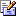Send e-mail